# Series and Parallel Circuits

In electronics, we can find both series and parallel circuits.

A series circuit is a circuit where the components are connected in a consecutive chain. This gives the current only one path to take.

A parallel circuit is a circuit where the components are connected parallel to each other. So the current will flow in several paths.

Often, real circuits have a mix of series and parallel circuits.

## Series Circuits

### Current in Circuits Connected in Series

The current in a series circuit is equal everywhere in the path. The same amount of current flows through all the components. To find the current use Ohm’s law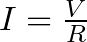### Resistance in Series Circuits

If you have several resistors connected in series, it’s very easy to find the total resistance. All you have to do is to find the sum of all the resistance values.### Capacitors Connected in Series

Capacitors connected in series are a little more complicated to calculate, but still easy once you learn it.

This is the formula for calculating capacitance values: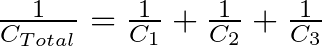To find CTOTAL you need to flip both sides, and you’ll end up with this: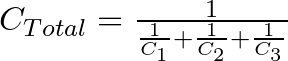## Parallel Circuits

Calculating resistance and capacitance in a parallel circuit is kind of funny. You just switch the methods from the series circuits =)

To calculate resistances in parallel you use the same method used to calculate capacitors in series. And vice versa.

### Current in Parallel Circuits

The current in parallel circuits can be different from path to path. To find the current of a path, you use Ohm’s law.### Calculating Resistors in Parallel

To calculate the resistance in a parallel circuit, you use the following formula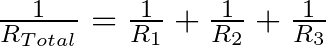To find RTOTAL you need to invert both sides, and you’ll end up with this: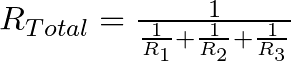### Calculating Capacitors in Parallel

You calculate the capacitance from capacitors in parallel simply by taking the sum of all the capacitance values.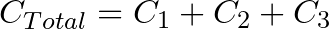## More Basic Electronics Tutorials

### 9 thoughts on “Series and Parallel Circuits”

1. Can you also please guide me on how to design simple circuits like dark sensors, etc….

2. ya i understand how resistor and capacitor work in parallel &series

thanks to provide me easy solution

• Great to hear buddy!

Oyvind

3. hi sir,

can i get how to design the inductor and transformer please suggest me.

thanks,